# Texas Go Math Grade 2 Lesson 10.5 Answer Key 3-Digit Subtraction: Regroup Tens

Refer to our Texas Go Math Grade 2 Answer Key Pdf to score good marks in the exams. Test yourself by practicing the problems from Texas Go Math Grade 2 Lesson 10.5 Answer Key 3-Digit Subtraction: Regroup Tens.

## Texas Go Math Grade 2 Lesson 10.5 Answer Key 3-Digit Subtraction: Regroup Tens

Explore

Use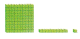to model the problem. Draw a quick picture to show what you did.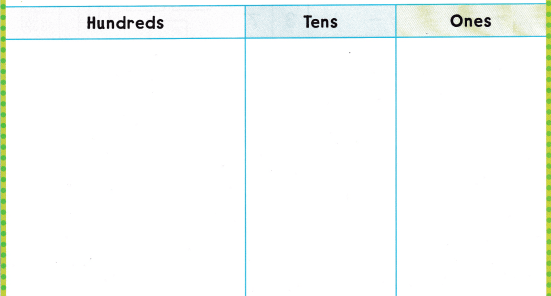FOR THE TEACHER • Read the following problem and have children model it with blocks. 473 people went to the football game 146 people were still there at the end of the game. How many people left before the end of the game? Have children draw quick pictures of their models.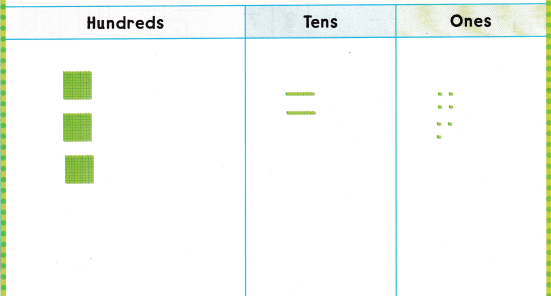Explanation:
The difference of 473 and 146 is 327.

Math Talk
Mathematical Processes

Describe what to do when there are not enough ones to subtract from.

Model and DrawShare and Show

Solve. Write the difference.

Question 1.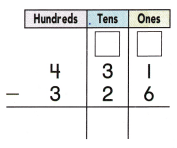Explanation:
The difference of 431 and 326 is 105.

Question 2.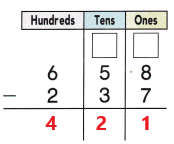Explanation:
The difference of 658 and 237 is 421.

Problem Solving

Solve. Write the difference.

Question 3.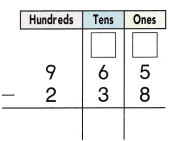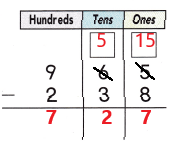Explanation:
The difference of 965 and 238 is 727.

Question 4.Explanation:
The difference of 489 and 149 is 340.

Question 5.Explanation:
The difference of 645 and 227 is 418.

Question 6.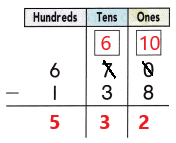Explanation:
The difference of 670 and 138 is 532.

Solve. Write or draw to explain.

Question 7.
H.O.T. Multi-Step There were 287 music books and 134 science books in the store. After some books were sold, there are 159 books left. How many books were sold?_________ booksExplanation:
The difference of 421 and 159 is 262.
262 books are sold.

Question 8.
H.O.T. There are 235 whistles and 42 bells in the store. Ryan counts 128 whistles on the shelf. How many whistles are not on the shelf?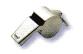_________ whistlesExplanation:
The difference of 235 and 128 is 107.
107 whistles are not on the shelf.

Question 9.
Analyze Harlan has 392 baseball cards. He gives Jack 115 cards. How many cards does Harlan have now?
(A) 347
(B) 277
(C) 237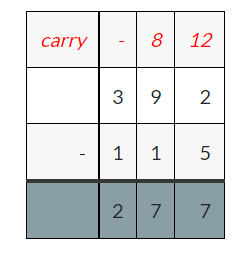Explanation:
The difference of 392 and 115 is 277.
Harlan have 277 baseball cards now.

Question 10.
Tywana has 291 soccer cards. She gives her brother 125 cards. How many cards does she have now?
(A) 115
(B) 125
(C) 166Explanation:
The difference of 291 and 125 is 166.
Tywana have 166 cards now.

Question 11.
Rey wants to collect all 196 cards of a set. So far, he has 157 of the cards. How many more cards does Rey need to complete the set?
(A) 49
(B) 39
(C) 41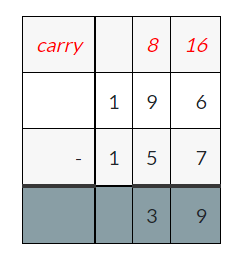Explanation:
The difference of 196 and 157 is 39.
Rey need 39 cards to complete the set.

Question 12.
TEXAS Test Prep Ms. Watson has 254 stickers. She gives 123 stickers to her students. How many stickers does she still have?(A) 121
(B) 137
(C) 131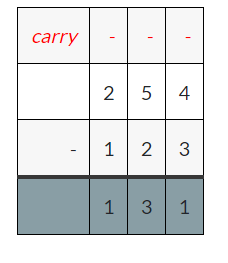Explanation:
The difference of 254 and 123 is 131.
Ms. Watson has 131 stickers now.

TAKE HOME ACTIVITY • Ask your child to explain why he or she regrouped in only some of the problems in this lesson.

### Texas Go Math Grade 2 Lesson 10.5 Homework and Practice Answer Key

Solve. Write the difference.

Question 1.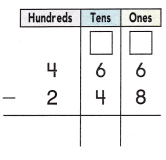Explanation:
The difference of 466 and 248 is 218.

Question 2.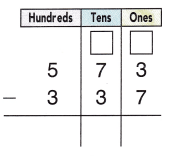Explanation:
The difference of 573 and 337 is 236.

Question 3.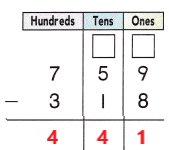Explanation:
The difference of 759 and 319 is 441.

Question 4.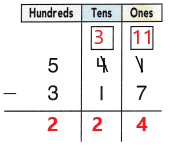Explanation:
The difference of 541 and 317 is 224.

Problem Solving

Solve. Write or draw to explain.

Question 5.
Multi-Step A bookstore has 148 books about people and 136 books about places. Some books were sold. Now there are 137 books left. How many books were sold?
___________ books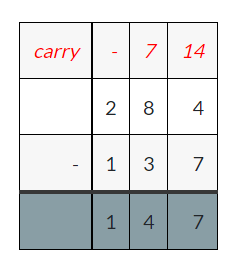Explanation:
The difference of 284 and 137 is 147.
147 books were sold.

Lesson Check

Question 6.
Ms. Bradley has 285 gold seals. She gives 68 gold seals to her students. How many gold seals does she still have?
(A) 223
(B) 227
(C) 217Explanation:
The difference of 285 and 68 is 217.
Ms. Bradley still have 217 gold seals.

Question 7.
There are 253 baseball bots and 145 balls in the sports store. There are 144 baseball bats in boxes. How many baseball bats are not in boxes?
(A) 111
(B) 109
(C) 108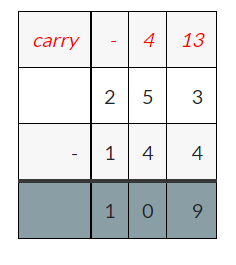Explanation:
The difference of 253 and 144 is 109.
109 baseball bats are not in boxes.

Question 8.
Lisa has 291 beads. She gives her cousin Sharon 125 beads to start a collection. How many beads does Lisa have left?
(A) 166
(B) 416
(C) 174Explanation:
The difference of 291 and 125 is 166.
166 beads are still left with Lisa.

Question 9.
Ryan wants to collect all 145 cars of a toy car collection. So far, he has 38 of the cars. How many more cars does Ryan need to complete the set?
(A) 183
(B) 113
(C) 107